# 求解薛定谔–泊松方程组的时间分裂紧致差分格式Time-Splitting Compact Difference Scheme for Solving Schrodinger-Poisson Equations

DOI: 10.12677/AAM.2019.81002, PDF, HTML, XML, 下载: 567  浏览: 1,872  国家自然科学基金支持

Abstract: In this paper, we have introduced fourth-order compact finite difference, the time splitting method and the Crank-Nicolson method to solve the nonlinear Schrödinger-Poisson equations. Based on fast Sine transform, we construct a fast solver for the fully discretized system. The presented numerical algorithm has been used to solve one-dimensional, two-dimensional and three-dimensional nonlinear Schrödinger-Poisson equations. We provide specific numerical examples. Through the MATLAB software, we write matlab programs based on the presented numerical algorithm, calcu-late approximated error and draw the approximated numerical solution. The numerical results prove that the presented algorithm has spectral accuracy in space direction. They also confirm its efficiency and stability.

1. 引言

1.1. 1.1. 问题背景

$i{\partial }_{t}\psi \left(x,t\right)=-\frac{1}{2}\Delta \psi +V\left(x\right)\psi +\varphi \left(x,t\right)\psi +\beta {|\psi |}^{\frac{4}{d}}\psi ,\text{\hspace{0.17em}}\text{\hspace{0.17em}}x\in {R}^{d},\text{\hspace{0.17em}}t>0,$ (1.1)

$\Delta \varphi \left(x,t\right)=-{|\psi |}^{2},\text{ }x\in {R}^{d},$ (1.2)

$\psi \left(x,t=0\right)={\varphi }_{0}\left(x\right),\text{ }x\in {R}^{d},$

$\underset{|x|\to \infty }{lim}|\psi \left(x,t\right)|=0,$ (1.3)

$V=V\left(x\right)$ 为外势函数，形式已知。ϕ为泊松位势，通常写成卷积形式。

$N\left(t\right)={\int }_{{ℝ}^{d}}{|\psi \left(x,t\right)|}^{2}\text{d}x,$ (1.4)

$P\left(t\right)=-i{\int }_{{ℝ}^{d}}\text{ }{\psi }^{*}\left(x,t\right)\nabla \psi \left(x,t\right)\text{d}x,$ (1.5)

$L\left(t\right)=-i{\int }_{{ℝ}^{d}}\text{ }{\psi }^{*}\left(x,t\right)x×\nabla \psi \left(x,t\right)\text{d}x,$ (1.6)

$E\left(t\right)={\int }_{{ℝ}^{d}}\left[\frac{1}{2}{|\nabla \psi |}^{2}+V{|\psi |}^{2}+\frac{{|\nabla \varphi |}^{2}}{2}+\frac{d}{2+d}{|\psi |}^{2+4/d}\right]\text{d}x.$ (1.7)

1.2. 紧致差分格式设计

${x}_{i}=a+ih\text{\hspace{0.17em}}\left(0 称为节点，记 ${u}_{i}:=u\left({x}_{i}\right)$${{u}^{\prime }}_{i}:=\left(\frac{\text{d}u}{\text{d}x}\right)\left({x}_{i}\right)$${{{u}^{\prime }}^{\prime }}_{i}:=\left(\frac{{\text{d}}^{2}u}{\text{d}{x}^{2}}\right)\left({x}_{i}\right)$${{{{u}^{\prime }}^{\prime }}^{\prime }}_{i}\text{\hspace{0.17em}}\text{\hspace{0.17em}}:=\left(\frac{{\text{d}}^{3}u}{\text{d}{x}^{3}}\right)\left({x}_{i}\right)$${{{{{u}^{\prime }}^{\prime }}^{\prime }}^{\prime }}_{i}\text{\hspace{0.17em}}\text{\hspace{0.17em}}\text{\hspace{0.17em}}:=\left(\frac{{\text{d}}^{4}u}{\text{d}{x}^{4}}\right)\left({x}_{i}\right)$ ，其中i与M是正整数，a与b是给定的区间端点。

$\begin{array}{l}\beta {{{u}^{\prime }}^{\prime }}_{i-2}+\alpha {{{u}^{\prime }}^{\prime }}_{i-1}+{{u}^{\prime }}_{i}+\alpha {{{u}^{\prime }}^{\prime }}_{i+1}+\beta {{{u}^{\prime }}^{\prime }}_{i+2}\\ =c\frac{{u}_{i+3}-2{u}_{i}+{u}_{i-3}}{9{h}^{2}}+b\frac{{u}_{i+2}-2{u}_{i}+{u}_{i-2}}{4{h}^{2}}+a\frac{{u}_{i+1}-2{u}_{i}+{u}_{i-1}}{{h}^{2}},\end{array}$ (1.8)

$\frac{1}{10}{{{u}^{\prime }}^{\prime }}_{i-1}+{{{u}^{\prime }}^{\prime }}_{i}+\frac{1}{10}{{{u}^{\prime }}^{\prime }}_{i+1}=\frac{6}{5}\cdot \frac{{u}_{i+1}-2{u}_{i}+{u}_{i-1}}{{h}^{2}}.$ (1.9)

$2{{{u}^{\prime }}^{\prime }}_{i-1}+11{{{u}^{\prime }}^{\prime }}_{i}+2{{{u}^{\prime }}^{\prime }}_{i+1}=\frac{3}{4}\cdot \frac{{u}_{i+2}-2{u}_{i}+{u}_{i-2}}{{h}^{2}}+12\cdot \frac{{u}_{i+1}-2{u}_{i}+{u}_{i-1}}{{h}^{2}}$ (1.10)

1.3. 一维的离散Sine变换与逆变换

${u}_{i}=\underset{k=1}{\overset{M-1}{\sum }}\text{ }{\stackrel{^}{u}}_{k}\mathrm{sin}\left(\frac{ik\text{π}}{M}\right),\text{ }i=1,2,\cdots ,M-1,$ (1.11)

${\stackrel{^}{u}}_{k}=\frac{2}{M}\underset{i=1}{\overset{M-1}{\sum }}{u}_{i}\mathrm{sin}\left(\frac{ik\text{π}}{M}\right),\text{ }k=1,2,\cdots ,M-1,$ (1.12)

${u}_{i+1}=\underset{k=1}{\overset{M-1}{\sum }}\text{ }{\stackrel{^}{u}}_{k}\mathrm{sin}\left(\frac{\left(i+1\right)k\text{π}}{M}\right),\text{ }{u}_{i-1}=\underset{k=1}{\overset{M-1}{\sum }}\text{ }{\stackrel{^}{u}}_{k}\mathrm{sin}\left(\frac{\left(i-1\right)k\text{π}}{M}\right),$

${{{u}^{\prime }}^{\prime }}_{i}=\underset{k=1}{\overset{M-1}{\sum }}{{{\stackrel{^}{u}}^{\prime }}^{\prime }}_{k}\mathrm{sin}\left(\frac{ik\text{π}}{M}\right),$ (1.13)

${{{u}^{\prime }}^{\prime }}_{i+1}=\underset{k=1}{\overset{M-1}{\sum }}{{{\stackrel{^}{u}}^{\prime }}^{\prime }}_{k}\mathrm{sin}\left(\frac{\left(i+1\right)k\text{π}}{M}\right),\text{ }{{{u}^{\prime }}^{\prime }}_{i-1}=\underset{k=1}{\overset{M-1}{\sum }}{{{\stackrel{^}{u}}^{\prime }}^{\prime }}_{k}\mathrm{sin}\left(\frac{\left(i-1\right)k\text{π}}{M}\right).$

$\begin{array}{l}\underset{k=1}{\overset{M-1}{\sum }}\text{ }{{{\stackrel{^}{u}}^{\prime }}^{\prime }}_{k}\left\{\frac{1}{10}\mathrm{sin}\left(\frac{\left(i-1\right)k\text{π}}{M}\right)+\mathrm{sin}\left(\frac{ik\text{π}}{M}\right)+\frac{1}{10}\mathrm{sin}\left(\frac{\left(i+1\right)k\text{π}}{M}\right)\right\}\\ =\frac{6}{5{h}^{2}}\underset{k=1}{\overset{M-1}{\sum }}\text{ }{\stackrel{^}{u}}_{k}\left\{\mathrm{sin}\left(\frac{\left(i-1\right)k\text{π}}{M}\right)+\mathrm{sin}\left(\frac{\left(i+1\right)k\text{π}}{M}\right)-2\mathrm{sin}\left(\frac{ik\text{π}}{M}\right)\right\}.\end{array}$

$\underset{k=1}{\overset{M-1}{\sum }}\text{ }{{{\stackrel{^}{u}}^{\prime }}^{\prime }}_{k}\left(\frac{1}{5}\mathrm{cos}\left(\frac{k\text{π}}{M}\right)+1\right)\mathrm{sin}\left(\frac{ik\text{π}}{M}\right)=\frac{6}{5{h}^{2}}\underset{k=1}{\overset{M-1}{\sum }}{\stackrel{^}{u}}_{k}\left(2\mathrm{cos}\left(\frac{k\text{π}}{M}\right)-2\right)\mathrm{sin}\left(\frac{ik\text{π}}{M}\right).$

${\stackrel{^}{u}}_{k}=-{{{\stackrel{^}{u}}^{\prime }}^{\prime }}_{k}{\left(\frac{24si{n}^{2}\left(\frac{k\text{π}}{2M}\right)}{{h}^{2}}\right)}^{-1}\left(cos\left(\frac{k\text{π}}{M}\right)+5\right),\text{\hspace{0.17em}}k=1,2,\cdots ,M-1.$ (1.14)

${\stackrel{^}{u}}_{k}=-{{{\stackrel{^}{u}}^{\prime }}^{\prime }}_{k}\frac{\frac{24}{{h}^{2}}\left(\mathrm{cos}\left(k\text{π}/M\right)-1\right)+\frac{6}{4{h}^{2}}\left(\mathrm{cos}\left(2k\text{π}/M\right)-1\right)}{4\mathrm{cos}\left(j\text{π}/M\right)+11},\text{\hspace{0.17em}}k=1,2,\cdots ,M-1.$ (1.15)

2. 快速高效的数值方法

2.1. 一维非线性薛定谔泊松方程组的求解方法

$i{\psi }_{t}=-\frac{1}{2}{\psi }_{xx}+V\left(x\right)\psi +\varphi \psi +\beta {|\psi |}^{2}\psi ,\text{\hspace{0.17em}}\text{\hspace{0.17em}}a (2.16)

$-{\varphi }_{xx}={|\psi |}^{2},$

$\psi \left(a,t\right)=0,\psi \left(b,t\right)=0,$ (2.17)

$\psi \left(x,0\right)={\varphi }_{0}\left(x\right),$

$-i{\stackrel{¯}{\psi }}_{t}\psi =V\left(x\right){|\psi \left(x,t\right)|}^{2}+\beta {|\psi \left(x,t\right)|}^{4}+\varphi {|\psi \left(x,t\right)|}^{2},$ (2.18)

$i{\psi }_{t}\left(x,t\right)=V\left(x\right)\psi \left(x,t\right)+\beta {|\psi \left(x,{t}_{n}\right)|}^{2}\psi \left(x,t\right)+\varphi \left(x,{t}_{n}\right)\psi \left(x,t\right),$ (2.19)

$i\frac{{\psi }_{t}}{\psi }=V\left(x\right)+\beta {|\psi \left(x,{t}_{n}\right)|}^{2}+\varphi \left(x,{t}_{n}\right),$ (2.20)

$\psi \left(x,{t}_{n+1}\right)=\psi \left(x,{t}_{n}\right){\text{e}}^{-i\tau \left(V\left(x\right)+\beta {|\psi \left(x,{t}_{n}\right)|}^{2}+\varphi \left(x,{t}_{n}\right)\right)},$ (2.21)

$\psi \left(x,{t}_{*}\right)=\psi \left(x,{t}_{n+1}\right)$$\psi \left(x,{t}_{*}\right)$ 作为时间分裂法的中间变量，但在方程(2.21)中，仍有未知量 $\varphi \left(x,{t}_{n}\right)$ 。由已知条件 $-\Delta \varphi ={|\psi |}^{2}$ ，即 $-{\varphi }_{xx}\left({x}_{j},{t}_{n}\right)={f}_{j}$ ，其中记 ${f}_{j}={|\psi \left({x}_{j},{t}_{n}\right)|}^{2}$ 。可先利用四阶紧致差分格式离散一维泊松方程 $-\Delta \varphi ={|\psi |}^{2}$ ，然后对离散后的方程组做Sine变换得 $-{\stackrel{^}{\varphi }}_{xx}={\stackrel{^}{f}}_{j}$ (详细过程见  )。该式子右边 ${\stackrel{^}{f}}_{j}$

${\stackrel{^}{\varphi }}_{j}^{xx}=-{\stackrel{^}{\varphi }}_{j}\left(\frac{24si{n}^{2}\left(\frac{j\text{π}}{2M}\right)}{{h}_{x}^{2}}\right){\left(cos\left(\frac{j\text{π}}{M}\right)+5\right)}^{-1},$

$-{\stackrel{^}{\varphi }}_{j}^{xx}={\stackrel{^}{f}}_{j},$

${\stackrel{^}{\varphi }}_{j}={\stackrel{^}{f}}_{j}{\left(\frac{24si{n}^{2}\left(\frac{j\text{π}}{2M}\right)}{{h}_{x}^{2}}\right)}^{-1}\left(cos\left(\frac{j\text{π}}{M}\right)+5\right),$ (2.22)

Crank-Nicolson公式离散：

$i\frac{{\psi }_{j}^{n+1}-{\psi }_{j}^{*}}{\tau }=-\frac{1}{4}\left({\left({\psi }_{j}^{*}\right)}_{xx}+{\left({\psi }_{j}^{n+1}\right)}_{xx}\right),$

$\left(1-\frac{i\tau }{4}\Delta \right){\psi }_{j}^{n+1}=\left(1+\frac{i\tau }{4}\Delta \right){\psi }_{j}^{*},$

${\psi }_{j}^{n+1}-\frac{i\tau }{4}{\left({\psi }_{j}^{n+1}\right)}_{xx}={\psi }_{j}^{*}+\frac{i\tau }{4}{\left({\psi }_{j}^{*}\right)}_{xx},$ (2.23)

${\left({\stackrel{^}{\psi }}_{j}^{*}\right)}_{xx}=-{\stackrel{^}{\psi }}_{j}^{*}\frac{24si{n}^{2}\left(\frac{j\text{π}}{2M}\right)}{{h}_{x}^{2}\left(cos\left(\frac{j\text{π}}{M}\right)+5\right)},$ (2.24)

${\left({\stackrel{^}{\psi }}_{j}^{*}\right)}_{xx}$ 。再做Sine变换就得 ${\left({\psi }_{j}^{*}\right)}_{xx}$ 。这样方程(2.23)的右边函数值全部已知，记 ${g}_{j}={\psi }_{j}^{*}+\frac{i\tau }{4}{\left({\psi }_{j}^{*}\right)}_{xx}$ ，对(2.23)两边同时做Sine变换 ${\stackrel{^}{\psi }}_{j}^{n+1}-\frac{i\tau }{4}{\left({\stackrel{^}{\psi }}_{j}^{n+1}\right)}_{xx}={\stackrel{^}{g}}_{j}$ ，其中 ${\stackrel{^}{g}}_{j}$${g}_{j}$ 经过一维的Sine逆变换得到的。对方程

(2.23)左边，我们利用基于Sine变换的四阶紧致差分格式的关系式：

${\left({\stackrel{^}{\psi }}_{j}^{n+1}\right)}_{xx}=-{\stackrel{^}{\psi }}_{j}^{n+1}\frac{24si{n}^{2}\left(\frac{j\text{π}}{2M}\right)}{{h}_{x}^{2}\left(cos\left(\frac{j\text{π}}{M}\right)+5\right)}$ (2.25)

${\stackrel{^}{\psi }}_{j}^{n+1}=\frac{{\stackrel{^}{g}}_{j}}{1+\frac{i\tau }{4}\frac{24si{n}^{2}\left(\frac{j\text{π}}{2M}\right)}{{h}_{x}^{2}\left(cos\left(\frac{j\text{π}}{M}\right)+5\right)}},$ (2.26)

1) 输入a，b，T，M，L，β，以及 ${\varphi }_{0}\left(x\right)$

2) 计算 ${x}_{j}=a+j{h}_{x}$$j=0,1,\cdots ,M$${t}_{n}=n\tau$${\psi }_{0}^{n}={\psi }_{M}^{n}=0$$n=0,1,\cdots ,L$ 。从 $n=0$ 出发；

3) 计算 ${f}_{j}={|{\psi }_{j}^{n}|}^{2}$ ，通过Sine逆变换得 ${\stackrel{^}{f}}_{j}$ ，利用 ${\stackrel{^}{\varphi }}_{j}^{n}={\stackrel{^}{f}}_{j}{\left(\frac{24si{n}^{2}\left(\frac{j\text{π}}{2M}\right)}{{h}_{x}^{2}}\right)}^{-1}\left(cos\left(\frac{j\text{π}}{M}\right)+5\right)$ ，再对 ${\stackrel{^}{\varphi }}_{j}^{n}$ 做Sine 变换得 ${\varphi }_{j}^{n}$

4) 由式子 ${\psi }_{j}^{*}={\psi }_{j}^{n}{\text{e}}^{-i\tau \left(V\left({x}_{j}\right)+\beta {|\psi \left({x}_{j},{t}_{n}\right)|}^{2}+{\varphi }_{j}^{n}\right)}$ ，计算出 ${\psi }_{j}^{*}$ ( $j=0,1,\cdots ,M$ )；

5) 通过Sine逆变换求出 ${\stackrel{^}{\psi }}_{j}^{*}$ ，再利用关系式(2.24)，求出 ${\left({\stackrel{^}{\psi }}_{j}^{*}\right)}_{xx}$ 。利用Sine变换求出 ${\psi }_{j}^{*}{}_{xx}$ ，再从 ${g}_{j}$ 的Sine逆变换求出 ${\stackrel{^}{g}}_{j}$

6) 由 ${\stackrel{^}{\psi }}_{j}^{n+1}=\frac{{\stackrel{^}{g}}_{j}}{1+\frac{i\tau }{4}\frac{24si{n}^{2}\left(\frac{j\text{π}}{2M}\right)}{{h}_{x}^{2}\left(cos\left(\frac{j\text{π}}{M}\right)+5\right)}}$ 得到 ${\stackrel{^}{\psi }}_{j}^{n+1}$ ，再对其做一次Sine变换就可得到 ${\psi }_{j}^{n+1}$ 。再令 $n=n+\text{1}$ ，重复3~6直到 $n=L$ 为止。

2.2. 二维非线性薛定谔泊松方程组的求解方法

$i{\psi }_{t}=-\frac{1}{2}\Delta \psi +V\psi +\beta {|\psi |}^{2}\psi +\varphi \left(x,y,t\right)\psi ,\text{\hspace{0.17em}}a0,$ (2.27)

$-\Delta \varphi \left(x,y,t\right)={|\psi |}^{2},$ (2.28)

$\psi \left(a,y,t\right)=0;\psi \left(b,y,t\right)=0,$

$\psi \left(x,c,t\right)=0;\psi \left(x,d,t\right)=0,$

$\psi \left(x,y,0\right)={\varphi }_{0}\left(x,y\right),$

${u}_{ij}=\underset{k=1}{\overset{M-1}{\sum }}\text{\hspace{0.17em}}\underset{l=1}{\overset{N-1}{\sum }}\text{\hspace{0.17em}}{\stackrel{^}{u}}_{kl}\mathrm{sin}\left(\frac{ik\text{π}}{M}\right)\mathrm{sin}\left(\frac{jl\text{π}}{N}\right),\text{\hspace{0.17em}}1\le i\le M-1,\text{\hspace{0.17em}}1\le j\le N-1,$ (2.29)

${\stackrel{^}{u}}_{kl}=\frac{2}{M}\frac{2}{N}\underset{i=1}{\overset{M-1}{\sum }}\text{\hspace{0.17em}}\underset{j=1}{\overset{N-1}{\sum }}\text{\hspace{0.17em}}{u}_{ij}\mathrm{sin}\left(\frac{ik\text{π}}{M}\right)\mathrm{sin}\left(\frac{jl\text{π}}{N}\right),\text{\hspace{0.17em}}1\le k\le M-1,\text{\hspace{0.17em}}1\le l\le N-1.$ (2.30)

$\psi \left(x,y,{t}_{*}\right)=\psi \left(x,y,{t}_{n}\right){\text{e}}^{-i\tau \left(V\left(x,y\right)+\beta {|\psi \left(x,y,{t}_{n}\right)|}^{2}+\varphi \left(x,y,{t}_{n}\right)\right)},$ (2.31)

$\psi \left(x,y,{t}_{*}\right)$ 作为时间分裂法的中间变量，即为第二部分的初始值。但是，等式右边仍然有未知量 $\varphi \left(x,y,{t}_{n}\right)$ 。为了求解它，我们要利用方程 $-{\left({\varphi }_{jk}^{n}\right)}_{xx}-{\left({\varphi }_{jk}^{n}\right)}_{yy}={|{\psi }_{jk}^{n}|}^{2}$ ，记 ${f}_{jk}={|{\psi }_{jk}^{n}|}^{2}$ 。对于上式做二维的Sine变换得： $-{\left({\stackrel{^}{\psi }}_{jk}^{n}\right)}_{xx}-{\left({\stackrel{^}{\psi }}_{jk}^{n}\right)}_{yy}={\stackrel{^}{f}}_{jk}$ ，观察发现：等式右边可由已知函数 ${f}_{jk}$ 做二维的Sine逆变换求得；等式

${\left({\stackrel{^}{\varphi }}_{jk}^{n}\right)}_{xx}=-{\stackrel{^}{\varphi }}_{jk}^{n}\frac{24si{n}^{2}\left(\frac{j\text{π}}{2M}\right)}{{h}_{x}^{2}\left(cos\left(\frac{j\text{π}}{M}\right)+5\right)},1\le j\le M-1,$ (2.32)

${\left({\stackrel{^}{\varphi }}_{jk}^{n}\right)}_{yy}=-{\stackrel{^}{\varphi }}_{jk}^{n}\frac{24si{n}^{2}\left(\frac{k\text{π}}{2N}\right)}{{h}_{y}^{2}\left(cos\left(\frac{k\text{π}}{N}\right)+5\right)},1\le k\le N-1,$ (2.33)

${\stackrel{^}{\varphi }}_{jk}^{n}=\frac{{\stackrel{^}{f}}_{jk}}{\frac{24si{n}^{2}\left(\frac{j\text{π}}{2M}\right)}{{h}_{x}^{2}\left(cos\left(\frac{j\text{π}}{M}\right)+5\right)}+\frac{24si{n}^{2}\left(\frac{k\text{π}}{2N}\right)}{{h}_{y}^{2}\left(cos\left(\frac{k\text{π}}{N}\right)+5\right)}}.$ (2.34)

$i\frac{{\psi }_{jk}^{n+1}-{\psi }_{jk}^{*}}{\tau }=-\frac{1}{4}\left(\Delta \left({\psi }_{jk}^{*}\right)+\Delta \left({\psi }_{jk}^{n+1}\right)\right)$

$\left(1-\frac{i\tau }{4}\Delta \right){\psi }_{jk}^{n+1}=\left(1+\frac{i\tau }{4}\Delta \right){\psi }_{jk}^{*}$

${\psi }_{jk}^{n+1}-\frac{i\tau }{4}\Delta \left({\psi }_{jk}^{n+1}\right)={\psi }_{jk}^{*}+\frac{i\tau }{4}\Delta \left({\psi }_{jk}^{*}\right)$

${\psi }_{jk}^{n+1}-\frac{i\tau }{4}\left({\left({\psi }_{jk}^{n+1}\right)}_{xx}+{\left({\psi }_{jk}^{n+1}\right)}_{yy}\right)={\psi }_{jk}^{*}+\frac{i\tau }{4}\left({\left({\psi }_{jk}^{*}\right)}_{xx}+{\left({\psi }_{jk}^{*}\right)}_{yy}\right),$ (2.35)

${\stackrel{^}{\psi }}_{jk}^{n+1}-\frac{i\tau }{4}\left({\left({\stackrel{^}{\psi }}_{jk}^{n+1}\right)}_{xx}+{\left({\stackrel{^}{\psi }}_{jk}^{n+1}\right)}_{yy}\right)={\stackrel{^}{\psi }}_{jk}^{*}+\frac{i\tau }{4}\left({\left({\stackrel{^}{\psi }}_{jk}^{*}\right)}_{xx}+{\left({\stackrel{^}{\psi }}_{jk}^{*}\right)}_{yy}\right),$ (2.36)

${\left({\stackrel{^}{\psi }}_{jk}^{*}\right)}_{xx}={\stackrel{^}{\psi }}_{jk}^{*}\frac{12}{{\left({h}_{x}\right)}^{2}}\frac{cos\left(\frac{j\text{π}}{M}\right)-1}{cos\left(\frac{j\text{π}}{M}\right)+5},$ (2.37)

${\left({\stackrel{^}{\psi }}_{jk}^{*}\right)}_{yy}={\stackrel{^}{\psi }}_{jk}^{*}\frac{12}{{\left({h}_{y}\right)}^{2}}\frac{cos\left(\frac{k\text{π}}{N}\right)-1}{cos\left(\frac{k\text{π}}{N}\right)+5},$ (2.38)

${\left({\stackrel{^}{\psi }}_{jk}^{n+1}\right)}_{xx}={\stackrel{^}{\psi }}_{jk}^{n+1}\frac{12}{{\left({h}_{x}\right)}^{2}}\frac{cos\left(\frac{j\text{π}}{M}\right)-1}{cos\left(\frac{j\text{π}}{M}\right)+5},$ (2.39)

${\left({\stackrel{^}{\psi }}_{jk}^{n+1}\right)}_{yy}={\stackrel{^}{\psi }}_{jk}^{n+1}\frac{12}{{\left({h}_{y}\right)}^{2}}\frac{cos\left(\frac{k\text{π}}{N}\right)-1}{cos\left(\frac{k\text{π}}{N}\right)+5}.$ (2.40)

${\stackrel{^}{\psi }}_{jk}^{n+1}=\frac{{\stackrel{^}{g}}_{jk}}{1-\frac{i\tau }{4}\left(\frac{12\left(cos\left(\frac{j\text{π}}{M}\right)-1\right)}{{h}_{x}^{2}\left(cos\left(\frac{j\text{π}}{M}\right)+5\right)}+\frac{12\left(cos\left(\frac{k\text{π}}{N}\right)-1\right)}{{h}_{y}^{2}\left(cos\left(\frac{k\text{π}}{N}\right)+5\right)}\right)}.$ (2.41)

1) 输入a，b，c，d，T，M，N，L，β，以及 ${\varphi }_{0}\left(x,y\right)$${h}_{x}$${h}_{y}$$\tau$

2) 计算 ${x}_{j}=a+j{h}_{x}$$j=0,1,\cdots ,M$${y}_{k}=c+k{h}_{y}$$k=0,1,\cdots ,N$${t}_{n}=n\tau$${\psi }_{0k}^{n}={\psi }_{Mk}^{n}=0$${\psi }_{j0}^{n}={\psi }_{jN}^{n}=0$$n=0,1,\cdots ,L$ 。 从 $n=0$ 出发；

3) 计算 ${f}_{jk}={|{\psi }_{jk}^{n}|}^{2}$ ，通过二维的Sine逆变换得 ${\stackrel{^}{f}}_{jk}$ ，利用关系式：

${\stackrel{^}{\varphi }}_{jk}^{n}=\frac{{\stackrel{^}{f}}_{jk}}{-\frac{12\left(cos\left(\frac{j\text{π}}{M}\right)-1\right)}{{h}_{x}^{2}\left(cos\left(\frac{j\text{π}}{M}\right)+5\right)}-\frac{12\left(cos\left(\frac{k\text{π}}{N}\right)-1\right)}{{h}_{y}^{2}\left(cos\left(\frac{k\text{π}}{N}\right)+5\right)}},$ (2.42)

4) 由 ${\psi }_{jk}^{*}={\psi }_{jk}^{n}{\text{e}}^{-i\tau \left(V\left({x}_{j},{y}_{k}\right)+\beta {|\psi \left({x}_{j},{y}_{k},{t}_{n}\right)|}^{2}+{\varphi }_{jk}^{n}\right)}$ ，计算出 ${\psi }_{jk}^{*}$

5) 通过二维的Sine逆变换求出 ${\stackrel{^}{\psi }}_{jk}^{*}$ ，再利用关系式(2.39)，(2.40)，求出 ${\left({\stackrel{^}{\psi }}_{jk}^{*}\right)}_{xx}$${\left({\stackrel{^}{\psi }}_{jk}\right)}_{yy}$

6) 最后由关系式(2.41)，求出 ${\stackrel{^}{\psi }}_{jk}^{n+1}$ ，对其作二维的Sine变换得 ${\psi }_{jk}^{n+1}$ 。再令 $n=n+\text{1}$ ，重复3~6直到 $n=L$ 为止。

2.3. 三维非线性薛定谔泊松方程组的求解方法

$-i{\psi }_{t}=-\frac{1}{2}\Delta \psi +V\psi +\beta {|\psi |}^{2}+\varphi \psi ,$ (2.43)

$-\Delta \varphi \left(x,y,z,t\right)={|\psi |}^{2},$ (2.44)

$\psi \left(a,y,z,t\right)=\psi \left(b,y,z,t\right)=0,$

$\psi \left(x,c,z,t\right)=\psi \left(x,d,z,t\right)=0,$

$\psi \left(x,y,e,t\right)=\psi \left(x,y,f,t\right)=0,$

$\psi \left(x,y,z,0\right)={\varphi }_{0}\left(x,y,z\right),$

${u}_{ijp}=\underset{k=1}{\overset{M-1}{\sum }}\text{\hspace{0.17em}}\underset{l=1}{\overset{N-1}{\sum }}\text{\hspace{0.17em}}\underset{q=1}{\overset{P-1}{\sum }}\text{ }{\stackrel{^}{u}}_{klq}sin\left(\frac{ik\text{π}}{M}\right)sin\left(\frac{jl\text{π}}{N}\right)sin\left(\frac{qp\text{π}}{P}\right),$ (2.45)

${\stackrel{^}{u}}_{klq}=\frac{2}{M}\frac{2}{N}\frac{2}{P}\underset{i=1}{\overset{M-1}{\sum }}\text{\hspace{0.17em}}\underset{j=1}{\overset{N-1}{\sum }}\text{\hspace{0.17em}}\underset{p=1}{\overset{P-1}{\sum }}\text{ }{u}_{ijp}\mathrm{sin}\left(\frac{ik\text{π}}{M}\right)\mathrm{sin}\left(\frac{jl\text{π}}{N}\right)\mathrm{sin}\left(\frac{qp\text{π}}{P}\right),$ (2.46)

1) 考虑非线性部分： $i{\psi }_{t}=V\psi +\beta {|\psi |}^{2}\psi +\varphi \psi$ ，利用共轭变换与积分性质得：

$\psi \left(x,y,z,{t}_{*}\right)=\psi \left(x,y,z,{t}_{n}\right){\text{e}}^{-i\tau \left(V\left(x,y,z\right)+\beta {|\psi \left(x,y,z,{t}_{n}\right)|}^{2}+\varphi \left(x,y,z,{t}_{n}\right)\right)}.$ (2.47)

$-{\varphi }_{xx}-{\varphi }_{yy}-{\varphi }_{zz}={|{\psi }_{jkl}^{n}|}^{2},$

$-{\stackrel{^}{\varphi }}_{xx}-{\stackrel{^}{\varphi }}_{yy}-{\stackrel{^}{\varphi }}_{zz}={|{\stackrel{^}{\psi }}_{jkl}^{n}|}^{2},$ (2.48)

${\stackrel{^}{f}}_{jlk}^{n}={|{\varphi }_{jkl}^{n}|}^{2}$ ，由基于Sine变换的四阶紧差分格式有如下关系式：

${\left({\stackrel{^}{\psi }}_{jkl}^{n}\right)}_{xx}={\stackrel{^}{\psi }}_{jlk}^{n}\frac{12\left(cos\left(\frac{j\text{π}}{M}\right)-1\right)}{{h}_{x}^{2}\left(cos\left(\frac{j\text{π}}{M}\right)+5\right)},$

${\left({\stackrel{^}{\psi }}_{jkl}^{n}\right)}_{yy}={\stackrel{^}{\psi }}_{jlk}^{n}\frac{12\left(cos\left(\frac{k\text{π}}{N}\right)-1\right)}{{h}_{y}^{2}\left(cos\left(\frac{k\text{π}}{N}\right)+5\right)},$

${\left({\stackrel{^}{\psi }}_{jkl}^{n}\right)}_{zz}={\stackrel{^}{\psi }}_{jlk}^{n}\frac{12\left(cos\left(\frac{l\text{π}}{P}\right)-1\right)}{{h}_{z}^{2}\left(cos\left(\frac{l\text{π}}{P}\right)+5\right)}.$ (2.49)

${\stackrel{^}{\varphi }}_{jkl}^{n}=\frac{{\stackrel{^}{f}}_{jkl}^{n}}{-\left(\frac{12\left(cos\left(\frac{j\text{π}}{M}\right)-1\right)}{{h}_{x}^{2}\left(cos\left(\frac{j\text{π}}{M}\right)+5\right)}+\frac{12\left(cos\left(\frac{k\text{π}}{N}\right)-1\right)}{{h}_{y}^{2}\left(cos\left(\frac{k\text{π}}{N}\right)+5\right)}+\frac{12\left(cos\left(\frac{l\text{π}}{P}\right)-1\right)}{{h}_{z}^{2}\left(cos\left(\frac{l\text{π}}{P}\right)+5\right)}\right)}.$ (2.50)

2) 考虑线性部分方程的求解： $i{\psi }_{t}=-\frac{1}{2}\Delta \psi$ ，其中 ${\psi }_{jlk}^{*}$ 作为起始值，在时间方向上，采用crank-nicolson

$i\frac{{\psi }_{jkl}^{n+1}-{\psi }_{jkl}^{*}}{\tau }=-\frac{1}{4}\left(\Delta {\psi }_{jkl}^{n+1}+\Delta {\psi }_{jkl}^{*}\right),$

${\psi }_{jkl}^{n+1}-\frac{i\tau }{4}\Delta \left({\psi }_{jkl}^{n+1}\right)={\psi }_{jkl}^{*}+\frac{i\tau }{4}\Delta \left({\psi }_{jkl}^{*}\right),$

${\stackrel{^}{\psi }}_{jkl}^{n+1}-\frac{i\tau }{4}\left[{\left({\stackrel{^}{\psi }}_{jkl}^{n+1}\right)}_{xx}+{\left({\stackrel{^}{\psi }}_{jkl}^{n+1}\right)}_{yy}+{\left({\stackrel{^}{\psi }}_{jkl}^{n+1}\right)}_{zz}\right]={\stackrel{^}{g}}_{jkl},$ (2.51)

${\stackrel{^}{g}}_{jkl}={\stackrel{^}{\psi }}_{jkl}^{*}+\frac{i\tau }{4}\left[{\left({\stackrel{^}{\psi }}_{jkl}^{*}\right)}_{xx}+{\left({\stackrel{^}{\psi }}_{jkl}^{*}\right)}_{yy}+{\left({\stackrel{^}{\psi }}_{jkl}^{*}\right)}_{zz}\right].$ (2.52)

${\left({\stackrel{^}{\psi }}_{jkl}^{*}\right)}_{xx}={\stackrel{^}{\psi }}_{jkl}^{*}\frac{12\left(cos\left(\frac{j\text{π}}{M}\right)-1\right)}{{h}_{x}^{2}\left(cos\left(\frac{j\text{π}}{M}\right)+5\right)},$

${\left({\stackrel{^}{\psi }}_{jkl}^{*}\right)}_{yy}={\stackrel{^}{\psi }}_{jkl}^{*}\frac{12\left(cos\left(\frac{k\text{π}}{N}\right)-1\right)}{{h}_{y}^{2}\left(cos\left(\frac{k\text{π}}{N}\right)+5\right)},$

${\left({\stackrel{^}{\psi }}_{jkl}^{*}\right)}_{zz}={\stackrel{^}{\psi }}_{jkl}^{*}\frac{12\left(cos\left(\frac{l\text{π}}{P}\right)-1\right)}{{h}_{z}^{2}\left(cos\left(\frac{l\text{π}}{P}\right)+5\right)}.$

${\left({\stackrel{^}{\psi }}_{jkl}^{n+1}\right)}_{xx}={\stackrel{^}{\psi }}_{jkl}^{n+1}\frac{12\left(cos\left(\frac{j\text{π}}{M}\right)-1\right)}{{h}_{x}^{2}\left(cos\left(\frac{j\text{π}}{M}\right)+5\right)},$

${\left({\stackrel{^}{\psi }}_{jkl}^{n+1}\right)}_{yy}={\stackrel{^}{\psi }}_{jkl}^{n+1}\frac{12\left(cos\left(\frac{k\text{π}}{N}\right)-1\right)}{{h}_{y}^{2}\left(cos\left(\frac{k\text{π}}{N}\right)+5\right)},$

${\left({\stackrel{^}{\psi }}_{jkl}^{n+1}\right)}_{zz}={\stackrel{^}{\psi }}_{jkl}^{n+1}\frac{12\left(cos\left(\frac{l\text{π}}{P}\right)-1\right)}{{h}_{z}^{2}\left(cos\left(\frac{l\text{π}}{P}\right)+5\right)}.$

${\stackrel{^}{\psi }}_{jkl}^{n+1}=\frac{{\stackrel{^}{g}}_{jkl}}{1-\frac{i\tau }{4}\left(\frac{12\left(\mathrm{cos}\left(\frac{j\text{π}}{M}\right)-1\right)}{{h}_{x}^{2}\left(cos\left(\frac{j\text{π}}{M}\right)+5\right)}+\frac{12\left(\mathrm{cos}\left(\frac{k\text{π}}{N}\right)-1\right)}{{h}_{y}^{2}\left(cos\left(\frac{k\text{π}}{N}\right)+5\right)}+\frac{12\left(\mathrm{cos}\left(\frac{l\text{π}}{P}\right)-1\right)}{{h}_{z}^{2}\left(cos\left(\frac{l\text{π}}{P}\right)+5\right)}\right)},$ (2.53)

1) 输入a，b，c，d，M，N，P，L，e，f，T，β，以及 ${\varphi }_{0}\left(x,y,z\right)$ ，hx，hy，hz，τ；

2) 计算 ${x}_{j}=a+j{h}_{x}$$j=0,1,\cdots ,M$${y}_{k}=c+k{h}_{y}$$k=0,1,\cdots ,N$${z}_{l}=e+l{h}_{z}$$l=0,1,\cdots ,P$${t}_{n}=n\tau$${\psi }_{0kl}^{n}={\psi }_{Mkl}^{n}=0$${\psi }_{j0l}^{n}={\psi }_{jNl}^{n}=0$${\psi }_{jk0}^{n}={\psi }_{jkP}^{n}=0$ 。从 $n=0$ 出发；

3) 计算 ${f}_{jkl}={|{\psi }_{jkl}^{n}|}^{2}$ ，通过三维Sine逆变换得 ${\stackrel{^}{f}}_{jkl}$ ，利用关系式(2.50)求出 ${\stackrel{^}{\varphi }}_{jkl}^{n}$ ，再做三维Sine变换得 ${\varphi }_{jkl}^{n}$

4) 由 ${\psi }_{jkl}^{*}={\psi }_{jkl}^{n}{\text{e}}^{-i\tau \left(V\left({x}_{j},{y}_{k},{z}_{l}\right)+\beta {|\psi \left({x}_{j},{y}_{k},{z}_{l},{t}_{n}\right)|}^{2}+{\varphi }_{jkl}^{n}\right)}$ ，计算出 ${\psi }_{jkl}^{*}$

5) 通过三维Sine逆变换求出 ${\stackrel{^}{\psi }}_{jkl}^{*}$ ，利用 ${\stackrel{^}{\psi }}_{jkl}^{*}$${\left({\stackrel{^}{\psi }}_{jkl}^{*}\right)}_{xx}$${\left({\stackrel{^}{\psi }}_{jkl}^{*}\right)}_{xx}$${\left({\stackrel{^}{\psi }}_{jkl}^{*}\right)}_{xx}$ 的关系，求出(2.52)中的 ${\stackrel{^}{g}}_{jkl}$

6) 利用方程(2.53)得 ${\stackrel{^}{\psi }}_{jkl}^{n+1}$ ，对其做三维Sine变换得 ${\psi }_{jkl}^{n+1}$ 。再令 $n=n+\text{1}$ ，重复3~6直到 $n=L$ 为止。

3. 数值计算例子

3.1. 一维情形

$V=V\left(x\right)=\frac{1}{2}{x}^{2}$${\varphi }_{0}\left(x\right)=\frac{1}{{\left(2\text{π}\right)}^{\frac{1}{4}}}{\text{e}}^{\frac{-{x}^{2}}{4}}$ 。通过改变空间步长h的大小做误差分析，在计算中我们取M = 512或空间步长 $h=\frac{1}{32}$ 所得的近似解看作是方程的准确解 ${u}_{exact}\left(x,t\right)$ 。我们通过第2.1节中所介绍的一维问题算法来求解，编写的代码在matlab软件中进行演算。误差定义 $error=\underset{0\le j\le M}{\mathrm{max}}|u\left({x}_{j},{t}_{n}=1\right)-{u}_{exact}\left({x}_{j},{t}_{n}=1\right)|$

3.2. 二维情形

$V=V\left(x,y\right)=\frac{1}{2}\left({x}^{2}+{y}^{2}\right)$ ，初值函数为 ${\varphi }_{0}\left(x,y\right)=\frac{1}{\sqrt{2\text{π}}}{\text{e}}^{\frac{-\left({x}^{2}+{y}^{2}\right)}{4}}$ 。通过改变空间步长hx,hy的大小做误差分析，在计算中我们取 $M=N=\text{512}$ 或空间步长 ${h}_{x}={h}_{y}=\frac{1}{32}$ 所得的近似解看作是方程的准确解 ${u}_{exact}\left(x,y,t\right)$ 。我

$error=\underset{0\le j\le M,0\le k\le P}{\mathrm{max}}|u\left({x}_{j},{y}_{k},{t}_{n}=1\right)-{u}_{exact}\left({x}_{j},{y}_{k},{t}_{n}=1\right)|$Table 1. Error analysis of one-dimensional space calculation at time t = 1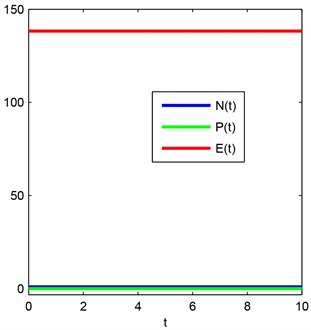Figure 1. The trend of the total number of particles N(t), impulse P(t), and total energy E(t) over time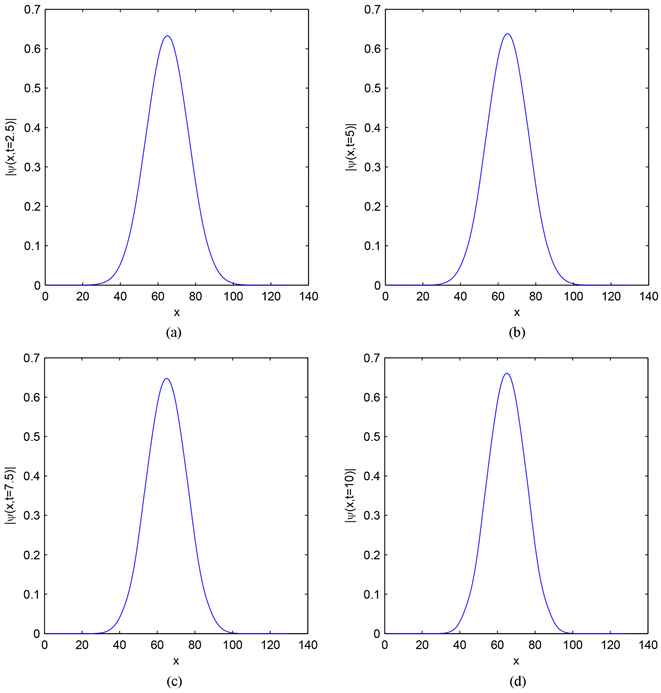Figure 2. Image of density function $|\psi \left(x,t\right)|$ at different times: (a) t = 2.5; (b) t = 5; (c) t = 7.5; (d) t = 10Table 2. Error analysis of two-dimensional space calculation at time t = 1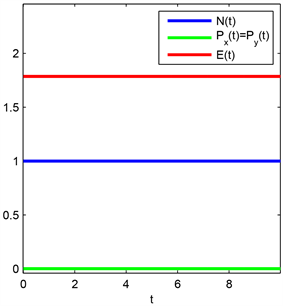Figure 3. The trend of the total number of particles N(t), the component of the impulse Px(t) Py(t), and the total energy E(t) over time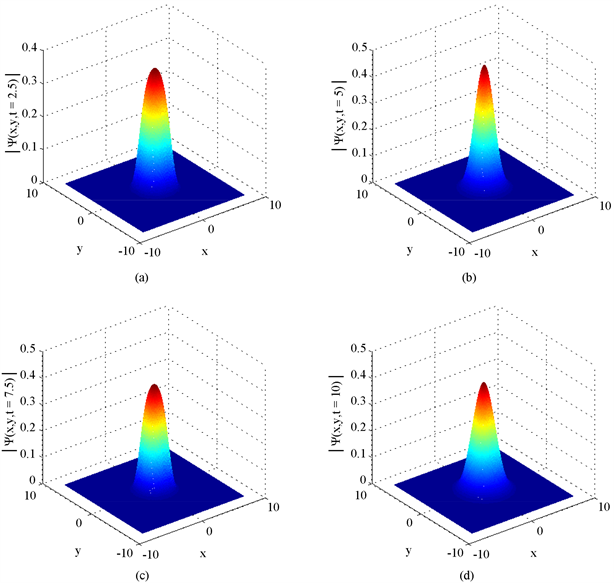Figure 4. Image of density function $|\psi \left(x,y,t\right)|$ at different times: (a) t = 2.5; (b) t = 5; (c) t = 7.5; (d) t = 10

3.3. 3.3. 三维情形

$V=V\left(x,y,z\right)=\frac{1}{2}\left({x}^{2}+{y}^{2}+{z}^{2}\right)$ ，初值函数为 ${\varphi }_{0}\left(x,y,z\right)=\frac{1}{2{\text{π}}^{3/4}}{\text{e}}^{\frac{-\left({x}^{2}+{y}^{2}+{z}^{2}\right)}{4}}$ 。通过改变空间步长hx，hy，hz的大小做误差分析，在计算中我们取 $M=N=P=512$ 或空间步长 $h=\frac{1}{32}$ ，所得的近似解看作是方程的准确解 ${u}_{exact}\left(x,y,z,t\right)$ 。我们通过2.2节中 所介绍的二维问题算法来求解， 编写的代码在matlab软件中进行演算。误差定义 $error=\underset{0\le j\le M,0\le k\le N,0\le l\le P}{\mathrm{max}}|u\left({x}_{j},{y}_{k},{z}_{l},{t}_{n}=1\right)-{u}_{exact}\left({x}_{j},{y}_{k},{z}_{l},{t}_{n}=1\right)|$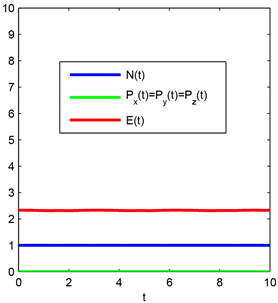Figure 5. The trend of the total number of particles N(t), the component of the impulse Px(t), Py(t), Pz(t), and the total energy E(t) over time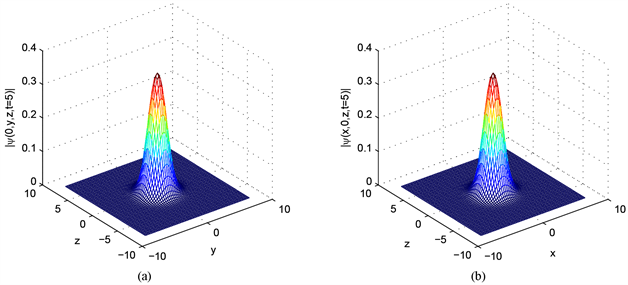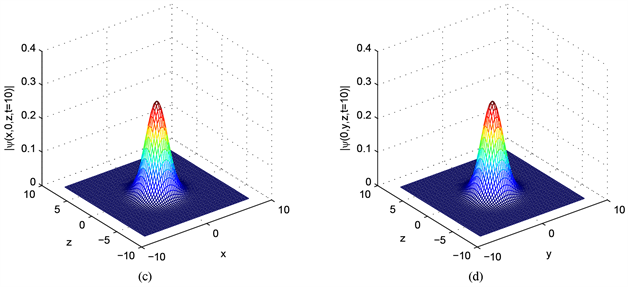Figure 6. Density function $|\psi \left(0,y,z,t\right)|$ images at different times: (a) t = 5, (c) t = 10; Density function $|\psi \left(x,0,z,t\right)|$ images at different times: (b) t = 5, (d) t = 10

4. 结论

  Anderson, D., et al. (2002) Statistical Effects in the Multistream Model for Quantum Plasmas. Physical Review E, 65, 046417. https://doi.org/10.1103/PhysRevE.65.046417  Ben Abdallah, N. (2000) On a Multidimensional Schrödinger-Poisson Scattering Model for Semiconductors. Journal of Mathematical Physics, 41, 4241-4261. https://doi.org/10.1063/1.533397  Shukla, P.K. and Stenflo, L. (2006) Stimulated Scattering Instabilities of Electromagnetic Waves in an Ultracold Quantum Plasma. Physics of Plasmas, 13, Article ID: 044505. https://doi.org/10.1063/1.2196248  Shukla, P.K. and Eliasson, B. (2007) Nonlinear Interactions between Electromagnetic Waves and Electron Plasma Oscillations in Quantum Plasmas. Physical Review Letters, 99, Article ID: 096401.  Ashcroft, N.W. and Mermin, N.D. (1976) Solid State Physics. Saunders College Publishing, Orlan-do.  Lange, H., Toomire, B. and Zweifel, P.F. (1995) An Overview of Schrödinger-Poisson Problems. Reports on Mathematical Physics, 36, 331-345. https://doi.org/10.1016/0034-4877(96)83629-9  Markowich, P.A., Ringhofer, C.A. and Schmeiser, C. (1990) Semiconductor Equations. Springer, Berlin. https://doi.org/10.1007/978-3-7091-6961-2  Davydov, A.S. (1979) Solitons in Molecular Systems. Physica Scripta, 20, 387-394. https://doi.org/10.1088/0031-8949/20/3-4/013  Cheng, C., Liu, Q., Lee, J. and Massoud, H.Z. (2004) Spectral Element Method for the Schrödinger-Poisson System. Journal of Computational Electronics, 3, 417-421. https://doi.org/10.1007/s10825-004-7088-z  Dong, X. (2011) A Short Note on Simplified Pseudospectral Methods for Computing Ground State and Dynamics of Spherically Symmetric Schrödinger-Poisson-Slater System. Journal of Computational Physics, 230, 7917-7922. https://doi.org/10.1016/j.jcp.2011.07.026  Shaikh, D. and Shukla, P.K. (2008) 3D Electron Fluid Turbulence at Nanoscales in Dense Plasmas. New Journal of Physics, 10, Article ID: 083007. https://doi.org/10.1088/1367-2630/10/8/083007  Mauser, N.J. and Zhang, Y. (2014) Exact Artificial Boundary Condition for the Poisson Equation in the Simulation of the 2D Schrödinger-Poisson System. Computer Physics Communications, 16, 764-780. https://doi.org/10.4208/cicp.110813.140314a  Moroz, I., Penrose, R. and Tod, P. (1998) Spherical-ly-Symmetric Solutions of the Schrödinger-Newton Equations. Classical and Quantum Gravity, 15, C2733-C2742. https://doi.org/10.1088/0264-9381/15/9/019  Brezzi, F. and Markowich, P.A. (1991) The Three-Dimensional Wigner-Poisson Problem: Existence, Uniqueness and Approximation. Mathematical Methods in the Applied Sciences, 14, 35-61. https://doi.org/10.1002/mma.1670140103  Castella, F. (1997) L2 Solutions to the Schrödinger-Poisson System: Existence, Uniqueness, Time Behavior, and Smoothing Effects. Mathematical Models and Methods in Applied Sciences, 7, 1051-1083. https://doi.org/10.1142/S0218202597000530  Bao, W. and Cai, Y. (2013) Mathematical Theory and Numerical Methods for Bose-Einstein Condensation. Kinetic and Related Models, 6, 1-135. https://doi.org/10.3934/krm.2013.6.1  Bao, W., Mauser, N.J. and Stimming, H.P. (2003) Effective One Particle Quantum Dynamics of Electrons: A Numerical Study of the Schrödinger-Poisson- Model. Communications in Mathematical Sciences, 1, 809-831. https://doi.org/10.4310/CMS.2003.v1.n4.a8  Haas, F., Manfredi, G. and Feix, M. (2000) Multistream Model for Quantum Plasmas. Physical Review B, 62, 2763. https://doi.org/10.1103/PhysRevE.62.2763  Manfredi, G., Haas, F. (2001) Self-Consistent Fluid Model for a Quantum Electron Gas. Physical Review B, 64, 075316. https://doi.org/10.1103/PhysRevB.64.075316  王芳芳. 紧致差分格式的理论及其分析[D]: [硕士学位论文]. 沈阳: 东北大学, 2010: 5-17.  Zhang, Y. (2013) Optimal Error Estimates of Compact Finite Difference Discretizations for the Schrödinger-Poisson System. Communications in Computational Physics, 13, 1357-1388. https://doi.org/10.4208/cicp.251011.270412a  张勇. 薛定谔–泊松方程组的数值计算和分析及其应用[D]: [博士学位论文]. 北京: 清华大学, 2012: 2-23.  Lele, S. (1992) Compact Finite Difference Scheme with Spectral-Like Resolution. Journal of Computational Physics, 103, 16-42.  Wang, Y. and Lu, X. (2014) Modulational Instability of Electrostatic Acoustic Waves in an Electron-Hole Semiconductor Quantum Plasma. Physics of Plasmas, 21, Article ID: 022107. https://doi.org/10.1063/1.4864329  Wang, H., Zhang, Y., Ma, X., Qiu, J. and Liang, Y. (2016) An Efficient Implementation of Fourth-Order Compact Finite Difference Scheme for Poisson Equation with Dirichlet Boundary Conditions. Computers & Mathematics with Applications, 71, C1843-C1860. https://doi.org/10.1016/j.camwa.2016.02.022